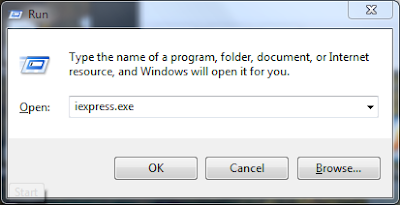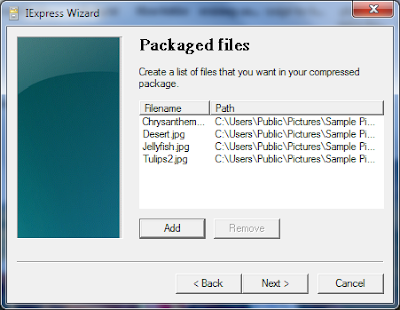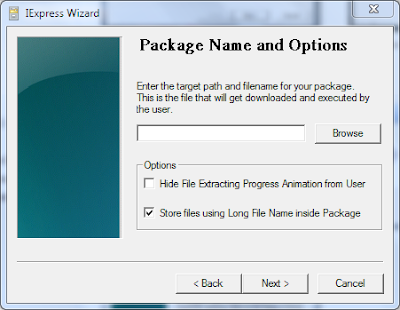## Tuesday, December 20, 2011

### C Program to Perform First Come First Serve Process Sheduling

First Come First serve is a Processes scheduling algorithm in which the process which arrives first gets System Resources First.The process with the least arrival time gets the system system resources first followed by processes with higher arrival time.

Waiting Time:
The Time Process has to wait in the ready list to get the system resources for its execution

Execution Time:
The time the process requires to perform the computation.

Turn Around Time:
The Total Time the Process Requires for completion waiting time+Execution Time

The following is a C Program Source Code of a First Come First Serve algorithm implementation.The processes are scheduled according to the arrival time and finally the waiting time and turn around time of the processes are calculated.

`````` #include<stdio.h>
void main()
{
int i,ex,tr,wt,n,ar;
int j,k,temp,od;
float b=0,c=0;
printf("\n Enter the number of processes : ");
scanf("%d",&n);
for(i=0;i<n;i++)
{
printf(" P%d = ",i+1);
printf("\n Excecution time : ");
scanf(" %d",&ex[i]);
printf("\n Arrival time : ");
scanf("%d",&ar[i]);
}
for(i=0;i<n;i++)
od[i]=ar[i];
for(i=0;i<n;i++)
for(j=i+1;j<n;j++)
{
if(ar[i]>ar[j])
{
temp=ar[i];
ar[i]=ar[j];
ar[j]=temp;
}
}
for(j=0;j<n;j++)
{
for(i=0;i<n;i++)
{
if(ar[j]==od[i])
{
if(j==0)
{
wt[i]=ar[j];
b=wt[i];
tr[i]=ex[i];
c=tr[i];
break;
}
wt[i]=temp-ar[j]+ar[j-1];
b=b+wt[i];
tr[i]=ex[i]+wt[i];
c=c+tr[i];
break;
}
}
temp=tr[i];
}
b=b/n;
c=c/n;
printf("\n ORDER NAME ARRIVAL-TIME EX.TIME WAITING-TIME TURN-AROUND-TIME \n");
for(j=0;j<n;j++)
for(i=0;i<n;i++)
{
if(ar[j]==od[i])
{
printf("  %d  P%d\t %d      %d\t   %d\t\t  %d ",j+1,i+1,ar[j],ex[i],wt[i],tr[i]);
printf("\n");
break;
}
}
printf("Average waiting time = %f \n Average turn around time = %f \n",b,c);
}
``````

## Saturday, December 10, 2011

### What is Abstraction?The Concept of Abstraction Simplified.

What is Abstraction in Object Oriented Programming?
Abstraction can be formally defined as the representation of an object including the specification of that object in a certain context.Abstraction is a powerful means to tackle the complexity of programming by wrapping up and thus reducing the complexity of complex operations.

Abstraction is used in OOP(Object Oriented Programming) Languages like Java,C++ etc.These OOP Languages employ an abstract entity called class from which instance of that class called object can be created.Data and Code are Binded together in a syntactic container called Object.

These statements can be little confusing for beginner's so read the rest of the post to get a better view of abstraction.

Abstraction Defined in a Simple way :

The concept of abstraction is a little confusing of beginner's.

To understand the concept of abstraction easily consider a cactus which has lots of spines placed in box.You can easily carry the box without knowing that a cactus is in it.But it is difficult to carry the cactus with bare hands.Thus Abstraction serves as a box to wrap up the complexities.

In programming subprograms are examples of abstractions,as they can be used to perform a function without knowing the internal details.eg: a function named add(int a,int b) can be used to add two integers and produce a result by simply using the code add(1,2) in the program.The internal details of the function that performs addition is not known to the programmer or to the main program.These details are abstracted and this provides a higher level view.

Abstraction simplifies programming as the programmer needs to focus only on the task he has to perform rather that the internal details of the program.Abstraction are provided in Libraries(.dll) for different operations.

Abstraction is implemented commonly in programming languages by using an data type called class.Objects are Instances of a class

eg:Car is a class Audi,Mercedes etc are Objects of the class named car

Each Object is an a clone of the an abstraction(class),it posses all the traits of class eg:Mercedes and Audi are two brands of cars but they posses all the characters of a the main class car.Thus they are Objects created from the Abstraction Car.The term Car is used to Abstract or wrap up the internal details like engine,wheels,brakes etc in a single container  named car.

Data Hiding is a Concept of Abstraction by which the Code and the Data in an Object(Instance of an Abstract Data type usually referred to as class) is hidden from the external entities.

## Saturday, December 3, 2011

### Round Robin Process Sheduling Algorithm and Example Source Code in C

Round robin is the most widely used process scheduling algorithm .The basic strategy for round robin scheduling is that if there are n process,each of the process will receive 1/n CPU Execution Time.Each process is allotted a  time quanta, for which its is executed.The incoming processes are kept in a ready list while another one is executing.If the time quanta allotted for a process is over,then that process is moved to ready and the next process in the ready list is executed for the allotted time quanta.The Complete Example Implementation Source Code in C of Round Robin Algorithm to schedule N Processes and to calculate the Execution,wait time and turn around time is given below.
`````` #include<stdio.h>
void main()
{
int n,i,k,x=0,s=0,r=0,q=0,x=0,a,e,t;
float m,p=0;
printf("Enter the number of process:");
scanf("%d",&n);
printf("Enter the execution time:");
for(i=0;i<n;i++)
{
scanf("%d",&a[i]);
e[i]=a[i];
}
printf("Enter the quanta:");
scanf("%d",&q);
printf("After round robin sheduling:");
for(i=0;i<n;i++)
{
if(x<a[i])
{
x=a[i];
}
}
k=x/q;
while(s<=k)
{
for(i=0;i<n;i++)
{
if(a[i]>0)
{
if(a[i]>q)
{
r=r+q;
a[i]=a[i]-q;
printf("P%d\t",i+1);
}
else
{
r=r+a[i];
a[i]=a[i]-q;
printf("P%d ",i+1);
t[i]=r;
}
}
}
s++;
}
printf("\nPROCESS EXECUTION TIME  WAIT TIME  TURN AROUND TIME\n");
for(i=0;i<n;i++)
{
printf(" %d \t\t %d\t\t %d\t\t %d\t\t \n",i,e[i],x,t[i]);
x=x+q;
}
m=x/n;
printf("Average waiting time=%f",m);
printf("Average turn around time=");
for(i=0;i<n;i++)
p=p+t[i];
p=p/n;
printf("%f",p);
}
``````

## Thursday, December 1, 2011

### How to create Self Extracting Executable using iexpress wizard

Windows has an inbuilt utility called iexpress that can be used to create self extracting archives or executables.IEXPRESS is used for the creation and distribution of self extracting exectables or setups or Packages.
Iexpress can be used easily to create your own setup or self extracting file that extracts and runs the desired program .Following is a step by step instructions for creating self extracting executable using the iexpress wizard.

• Go to run type in iexpress.exe• The iexpress wizard will open up,where you need to configure your setup or self extracting executable

• Select create a new self extracting directive as you are creating a new self extracting archive once your setup is configured you can save the SED( self extracting directive)• Now select the type of Self Extracting Executable from the next iexpress windows,Select the Option 'Extract Files Only'.You can try out other options later.• Choose if you want to display a license before the installation,This will be shown before the installation begins.Text files can be used for this purpose.• Then add the required files that should be installed or extracted by the self extracting executable.I add some photos then proceed to  the next step.• In the next Window select the style of the setup• Choose the Path to save the Self extracting executable or the Package that you are about to create• Choose if you want to save the configuration as a SED file for future use

• All Done!Open your own self extracting executable created with iexpress### Which is the Best Photo Watermarking Software

Photo Theft is becoming more and more common in the web with the outburst of social websites like Facebook,Google Plus and Image sharing se...